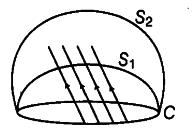# Consider a closed loop C in a magnetic field as shown in the figure

Consider a closed loop C in a magnetic field as shown in the figure. The flux passing through the loop is defined by choosing a surface whose edge coincides with the loop and using the formula Now, if we choose two different surfaces having C as their edge, would we get the same answer for flux. Justify your answer.The magnetic flux linked with the surface can be considered as the number of magnetic field lines passing through the surface. So, let d$\phi$ = BA represents magnetic lines in an area A and B.
By the concept of continuity of magnetic field lines, it cannot end or start in space, therefore the number of lines passing through surface ${ S }{ 1 }$
must be the same as the number of lines passing through the surface ${ S } { 2 }$. Therefore, in both the cases, we get the same answer for flux.